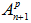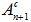# Adjustment of the time step: operation

## Introduction

The time step adjustment function enables the user to manage the choice of time step automatically in Flux. The user only has to specify a variation interval within which the time step will be able to vary.

The adjustment of the time step permits detection, with a high degree of accuracy, physical discontinuities such as :

• torque computation in a system with springs
• the using of controlled switches (switching times)
• a discontinued heat source (different phases of heating and cooling)
• the using of model depending of temperature with Gauss phenomena around the Curie temperature

## Principle of operation

The algorithm of prediction correction of the time step permits automatic adaption of the value of the time step during the solving process. It permits the adjustment of the time step in function of the variations of the state variable (the unknown of the problem). The operation principle is described in the table below.

Stage Description
1

Flux predicts the value of the state variable () starting from the results of two previous time steps (An and An-1)

2

Flux compares the predicted value to the actually computed value ()

3

If the difference between these values is:

• too low, Flux will increase the size of the time step
• too big, Flux will diminish the size of the time step

The maximum number of iteration is set at 5.

The limits: Flux can decrease/increase the value of the time step within the limits set by the user: minimal value/maximal value of the time step.

Step Action
1 Create a solving scenario: scenario_adjust
2

Set the bounds of the solving interval:

• lower limit
• higher limit
3

Choose the variation method: Adaptive time step

4

Set the following values:

• Initial value of the time step
• Minimal value of the time step
• Maximal value of the time step

## Precautions in operation (inferior limit)

During the computation, the algorithm diminishes the value of the time step as many times as necessary to obtain a minimal difference between the predicted value and the computed value.

The number of iterations is limited to 5. In the case where the algorithm reaches the minimal values specified by the user, the last time step is retained. The computation then passes to the next time step and the solving process continues normally.

Thus, in the case of MT coupled circuit and/or mechanical coupling problems, it is advisable to choose a minimal time step low enough so that the problem can be solved under the proper conditions. The computation time can be longer however.

As a reminder, for problems with solid conductor crossed by induced current, the user must be very cautious when choosing the time step.

## Precautions in use (higher limit)

If the higher limit of the time step is too big, certain physical phenomena can be masked as presented in the figure below.